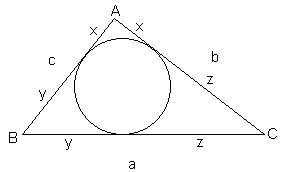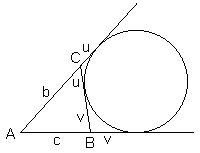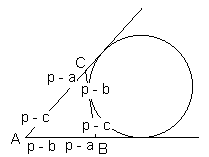# Incircles and Excircles in a Triangle

The points of tangency of the incircle of $\Delta ABC$ with sides $a,$ $b,$ $c,$ and semiperimeter $p = (a + b + c)/2,$ define the cevians that meet at the Gergonne point of the triangle. This follows immediately from Ceva's theorem and the fact that two tangents to a circle from a point outside the circle are equal.The length of those tangents from the vertices of the triangle to its incircle can be easily determined. Denote them $x,$ $y,$ $z,$ as in the diagram. We have three equations:

 (1) \begin{align} x + y &= c \\ y + z &= a \\ z + x &= b, \end{align}

from where

 (2) $x + y + z = p.$

Subtracting from (2) equations (1) one at a time, we get

 (3) \begin{align} x &= p - a\\ y &= p - b \\ z &= p - c. \end{align}

These are the lengths that appear in Heron's formula, e.g., $p - a = (b + c - a)/2.$Similarly we can find the lengths of the tangents to the excircles.Obviously, $2p = (b + u) + (c + v).$ But, since $(b + u) = (c + v),$ we get

 (4) \begin{align} u &= p - b \\ v &= p - c. \end{align}From the just derived formulas it follows that the points of tangency of the incircle and an excircle with a side of a triangle are symmetric with respect to the midpoint of the side. Such points are called isotomic. The cevians joinging the two points to the opposite vertex are also said to be isotomic. Both triples of cevians meet in a point. For the incircle, the point is Gergonne'; for the points of excircle tangency, the point is Nagel's. We have just proved that, in any triangle, the Gergonne and Nagel points are isotomic conjugate of each other. (This fact has an interesting geometric illustration.)

In general, two points in a triangle are isotomic conjugate if the cevians through them are pairwise isotomic. The centroid is one point that is its own isotomic conjugate.### cot(A/2) = (p - a)/r

This obvious formula sometimes goes under the name of The Law of Cotangents:

$\displaystyle\frac{\cot (A/2)}{p-a}=\frac{\cot (B/2)}{p-b}=\frac{\cot (C/2)}{p-c}=\frac{1}{r}.$### S = ra(p - a)

Indeed,\begin{align} 2S &= (b + u)r_{a} + (c + v)r_{a} - ar_{a} - (u + v)r_{a} \\ &= (b + c - a)r_{a} \\ &= (2p - 2a)r_{a}\\ &= 2(p - a)r_{a}. \end{align}•